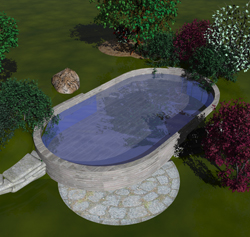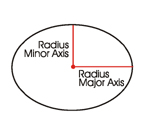# Pond Volume and Square Footage – Simple Calculations You Need To Know – Part 3 Ellipses

February 21, 2008CalculationsAn ellipse?  What the heck is an ellipse?  Simple.  Ellipses are circles.  Ok, did that confuse you?  Think of ellipses as special instance of a circle, meaning if you have an oval shape, it’s still considered a circle, but a special case of a circle.

If you have an oval shaped pond, you need to perform calculations for ellipses in order to figure out square footage and volume.  So, when we spoke about circles and some of your calculations were based off of the circle’s radius, it will be the same with an ellipse, but we’ll use the radius measurement just a little differently.

Major axis – The measurement that consists of the longest radius length from the center of the ellipse to the outer edge.

Minor axis –  The measurement that consists of the shortest radius length from the center of the ellipse to the outer edge.

Take a look at these pictures:With an oval shaped ellipse, you will have two radius measurements.  One is longer and will be referred to as the major axis, while the other is shorter and will be referred to as the minor axis.  Remember when you calculated the area of a circle, you multiplied the radius by the radius and then by Pi?  This works the same.

For an ellipse, you multiply the major axis by the minor axis and then by Pi.  So if the pond was a 20 feet long and 10 feet wide oval shape, you would calculate,
20 (major axis) x 10 (minor axis) x 3.14 (Pi) = 628

Do not forget that you will have to measure the circumference (perimeter of the pond) in order to have the total square footage of the walls of the pond.  This is where it gets a little scary.  Because an oval is essentially a squashed circle, there are a lot of variables that would have to be taken into account in order to calculate the circumference.

First let me say that I will show you the varying calculations and you are free to follow along if math is your thing.  However, you may find that simply walking a tape measure around the perimeter of you pond is much easier.  Ok here we go for the mathematic enthusiast.

The first calculation you can do in order to find the circumference is an approximation only.  This means that you have to be sure that your major axis is no more than three times longer than the minor axis and your resulting calculation will be within 5% of being true.  That is the approximation part.  Your calculation could be as much as 5% incorrect.  But hey, let’s look at it anyways.

You are first going to multiply the major axis by itself and the minor axis by itself, and then add those two products together.  Then divide that answer by 2.  Take that total and divide it by the product of Pi multiplied by 2.
20 (major axis) x 2 = 400
10 (minor axis) x 2 = 200
So now you have 600 /2 = 300
Now, 3.14 (Pi) x2 = 6.28
The divide 300 by 6.28
30 / 6.28 = 47.77 feet

Now again, I stress that this formula is an approximation and can be off, up to 5%.  So let’s just assume you wanted a formula that calculated the circumference of the oval shape almost exactly.  Well all I am going to do is just show you the formula because it is nothing short of horrific!

Circumference = Pi(3.14) x (major axis + minor axis) / 4 x [ 3 x (1+L) + 1 / (1-L) ] And that’s assuming L = h/4 = (major axis – minor axis) x 2 / [2 x (major axis + minor axis)] x 2
As well as assuming h = (major axis – minor axis) x 2 / (major axis + minor axis) x 2

Now do you see why I say that it is probably just easier to walk a tape measure around your pond?  There is just no way I even care to calculate the circumference when it would take less time to physically measure it.  I certainly do not expect you to either.  So save yourself the time and headache and just tape measure the circumference.

Now that you have the circumference measurement, you need to multiply that by the height of the pond, like this (assuming the height or depth is 3 feet):
47.77 (circumference) x 3 (height) = 143.31 square feet

So the floor area square footage plus the wall square footage would be:
628 (floor area) + 143.31 (walls) = 771.31 total square feet

Again, to calculate how much Pond Shield epoxy you would need to coat this pond, you divide the total square footage by 60 square feet.  Remember 60 square feet is the amount of coverage a quart and a half kits gives you at a minimal thickness of 10 mils.
771.31 / 60 = 12.8 (rounded up to 13 total quart and a half kits)

To calculate volume of a oval (ellipse) shaped pond, you multiply Pi (3.14) by the major axis and multiply that by the minor axis and then multiply that by the height or depth and divide the total by 4.like this,
3.14 x 20 x 10 x 3 / 4 = 471 cubic feet

Then on to my obsession with water weight.  How much would that weigh?  3,956.4 pounds.  That is quite a bit of water for a small elliptical shaped pond.  Oh, I almost forgot.  Not that we have talked about elliptical shaped ponds, remember Part 2’s question at the end?  Have you figured it out yet?

I will see you when we discuss triangular shaped ponds in part 4.